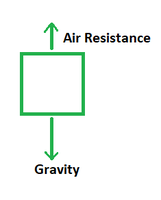Open In App

# Air Resistance Formula

Have you ever held your hand out of a speeding car or bus? The air pushes your hand in the direction opposite to the movement of the vehicle. An object falling down from say, a table slows down subsequently because a certain force slows down its fall by acting in the direction opposite to its motion. In both of these cases, a certain force is exerted by the atmosphere upon the objects, thereby slowing down their motion. The force and its formula are discussed below.

### Air Resistance

The force exerted by the air on things moving through it is known as air resistance. This force is commonly referred to by scientists as drag or drag force. Typically, this force is applied in the opposite direction as the object’s motion, slowing it down.

The frictional force of air resistance acts on the moving body. When a body moves, air resistance slows it down. The more the body’s motion, the greater the air resistance imposed on it. Air resistance affects all moving objects, including bicycles, automobiles, trains, rockets, airplanes, and even living bodies. As is evident from the picture below, the air resistance acts upon freely falling bodies too, in the direction opposite to the force of gravity.Examples

• Landing with a parachute: The air resistance force is particularly significant in the operation of a parachute. When a skydiver dives down and opens his or her parachute, the air resists the jump. The speed with which the parachute approaches the earth slows due to the resistance produced by the air. The gravity force pushes the parachute downward, while the air resistance force pulls the parachute upward. As a result, the air resistance force assists the individual in landing smoothly on the ground.
• Walking in a storm: Walking in inclement weather is frequently challenging. A significant degree of resistance is felt by the individual while walking in the direction of the wind, causing difficulties in walking. The same reason makes holding an umbrella in hand difficult in the face of a strong wind.
• Airplanes: An airplane’s engine, wings, and propellers are constructed in such a way that enough thrust can be created to assist the plane to overcome the air resistance force. Turbulence is also caused by the friction introduced by the air. However, air resistance does not pose a problem in the case of a rocket since a rocket must go in space, which is an environment devoid of air, and so is free of air resistance force.

Formula

The formula for air resistance is given as follows:

Fair = cv2

where,

• Fair depicts the force of air resistance
• c refers to the force constant
• v depicts the object’s velocity.

### Sample Problems

Question 1. Calculate the air resistance if an object moving at 50 ms-1 has a force constant of 0.05.

Solution:

Given: v = 50 ms-1 and c = 0.05

The formula for air resistance is Fair = cv2

Substitute the given values in the above formula. Then,

= (0.05)(50)2

Fair = 125 N

Question 2. Calculate the air resistance if an object moving at 40 ms-1 has a force constant of 0.08.

Solution:

Given: v = 40 ms-1 and c = 0.08

The formula for air resistance is Fair = cv2

Substitute the given values in the above formula. Then,

= (0.08)(40)2

Fair = 128 N

Question 3. Calculate the force constant if an object moving at 30 ms-1 experiences an air resistance of 20 N.

Solution:

Given: v = 30 ms-1 and Fair = 20 N

The formula for air resistance is Fair = cv2

Substitute the given values in the above formula. Then,

20 = c(30)2

c = 20/900

c = 0.023

Question 4. Calculate the force constant if an object moving at 20 ms-1 experiences an air resistance of 50 N.

Solution:

Given: v = 20 ms-1 and Fair = 50 N

The formula for air resistance is Fair = cv2

Substitute the given values in the above formula. Then,

50 = c(20)2

c = 50/400

c = 0.125

Question 5. Calculate the air resistance if an object moving at 30 ms-1 has a force constant of 0.02.

Solution:

Given: v = 30 ms-1 and c = 0.02

The formula for air resistance is Fair = cv2

Substitute the given values in the above formula. Then,

= (0.02)(30)2

Fair = 18 N

Question 6. Calculate the velocity of an object if its air resistance is 40 N and the force constant is 0.5.

Solution:

Given: Fair = 40 N and c = 0.5

The formula for air resistance is Fair = cv2

Substitute the given values in the above formula. Then,

v2 = Fair/c

v2 = 40/0.5

v2 = 80

v = 8.94 m/s

Question 7. Calculate the velocity of an object if its air resistance is 32 N and the force constant is 0.04.

Solution:

Given: Fair = 32 N and c = 0.04

The formula for air resistance is Fair = cv2

Substitute the given values in the above formula. Then,

v2 = Fair/c

v2 = 32/0.04

v2 = 800

v = 28.28 m/s